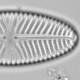## Sunspots and survival in Norway

```#load data
url<-"d://Norwegian%20Lifespan%20and%20birthyear.txt"

#remove births not in study window
ss2<-ss[ss\$Birth_Year>=1676&ss\$Birth_Year<=1878,]
nrow(ss2)#No births
nrow(ss)

#process data
n<-with(ss2, tapply(Lifespan, Birth_Year, function(x)length(x)))
surv<-with(ss2, tapply(Lifespan, Birth_Year, function(x)mean(x>=20)))
sun<-with(ss2, tapply(Solar, Birth_Year, function(x)x))
year<-(as.numeric(names(surv)))

#plot
x11(width=5, height=6);par(mar=c(3,3,1,3), mgp=c(1.5,.5,0))
plot(year, surv, col=ifelse(sun=="MIN", 2,1), ylab="",
xlab="Year CE", ylim=c(-0.4,1), yaxt="n")
axis(side=2, at=seq(0,1,.2))
title(ylab="Proportion surviving to age 20", adj=.7)
abline(v=year[sun=="MAX"], col="grey80", lwd=2)
#highlight solar maxima in grey
points(year, surv, col=ifelse(sun=="MIN", 2,1),
ylab="Proportion survival to age 20", xlab="", xaxt="n")
legend(1676, 0.2, c("Maximum","Minimum"), pch=1, col=1:2,
title="Solar")
par(new=TRUE)
plot(sunspot.year, xaxt="n", xlab="", xlim=range(year), yaxt="n",
ylab="",ylim=c(0, max(sunspot.year)*3))
axis(4, at=c(0,50,100))